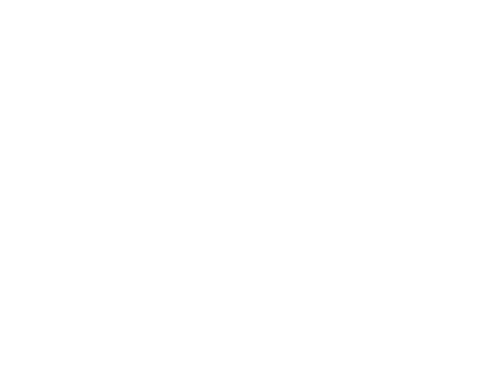# Rally X Final

Rally X - Final version . A comlete racing game written in only a few lines of (obfuscated) MSX-BASIC. Includes ranking & gear shifting.

Rally X1.31 KB9153 days 6 hours ago

Here is the source of this game:What's that @ at the end of line 6 doing there?

Here it is in ASCII:
{moderator says "no it's not"}

And here it is formatted with width 80 like the screenshot above:

```1 J=S-J*(J<96-S):K=J\16:FORW=0TO2:VPOKE8208+W,P(W+K):NEXT:IFTTHENRESUMENEXTELSEO
NERRORGOTO1:DEFINTA-Y:COLOR15,5,1:KEYOFF:SCREEN2,3,0:U=255:V=32:FORX=-1TO1:FORY=
0TO31:M=16-Y\2:LINE(M-X*((SIN(Y^.8/6)-(Y<16))*6-M-2)+(X<1),V+X*V+Y)-STEP(Y+1,0)
2 P(Y\4)=50-15*(Y\12AND1):NEXTY,X:N=14336:FORI=0TO383:VPOKEN+I,VPEEK((IAND7)+(IA
ND8)*V+(IAND48)\2+(IAND448)*8):NEXT:S\$="3##B#C#c\$B'r\$B&s3#%##C#3%S';%S&s;##2#3#B
#R#B#<=c'##c'#@C#)#2#,s##&wfw:w3w4w\$w3w#w4w,DD0CDDDS0CDSDK0CDKDC0CDC":SOUND3,14
3 SCREEN1:FORO=0TO53:Y=0:FORM=MTOM+1:Y=Y*96+(ASC(MID\$(S\$,M))-35):NEXT:FORK=KTOK+
Y\256:VPOKEK+N+I+8335*(O>V),YANDU:NEXTK,O:FORI=UTO1023:O=VPEEK(I):VPOKEI,(OORO\2
)\(2-(IAND4)\4):NEXT:FORI=ITO1279:O=IANDV:VPOKE6432+(IAND63),228+8*(IAND3)-O
4 W=2^(IAND7):J=O*U\W\64:VPOKEI+512,JAND-W:VPOKEI+768,JOR-WANDU:J=8-O\8:VPOKEI,(
JANDI)*U\J:NEXT:R=N\2:VPOKE8192,14:VPOKE1209,U:PLAY"v15o5arararo6aa":FORW=9TO10:
PUTSPRITEW,(16+64*W,159),14,W-3:SOUNDW,14:N=N+9:NEXT:TIME=0:SOUND5,83:FORT=2TOR
5 PUTSPRITE0,(X+80,156),1,12:G=G+F*S\2:LOCATE1,1:D=96-(NAND127):Y=D>0:D=D*D\96(:
PRINTUSING"SPEED:##0kmh  !  RANK:###";S;CHR\$(76-L*4);N\128-82(:FORW=8216TO8219:G
=G+8AND31:VPOKEW,86+(GAND16):VPOKE4+W,85+G\24:NEXT:@:Q=O:IFC99)*(1-CMOD3):L=(QANDO+Q)+LAND1:S=S+(0=(OAND6
4))-(0=(OANDV))*(2-((S>9)=-L)):S=(S>14+L*6)*(L+2)-(S>0)*(S-1)(:FORW=3TO4:PUTSPRI
TEW+1,(F*10+W*V,95),14,4*B+W+1:PUTSPRITE3+W,(W*V,127),14,4*F+3+W:NEXT:@
7 PUTSPRITE1,(115-(D<34)*((24-D)*B+F*10)+(E-64)*D\225,-Y*(98+D)-31),6,15-D\25-(T
>R)*9:M=(X>72)-(X<0):E=NAND128:N=N+9-S:S=S*((X-15-E\3)\15<>0ORD<73)*(M=0):SOUND4
>,U-S*9(:X=X+5*(M-(0=(OAND128))+(0=(OAND16)))-S*B\4:FORW=0TO0:W=PLAY(0):NEXTW,T
8 S=15:Z\$=SPACE\$(S)+"Race Over  Time ="+STR\$((TIME\(6-PEEK(43)\128))/10)+"s":FOR
N=0TOU:D=88-3*N:LOCATE6,4:PUTSPRITE0,(116-(36-X)*D\126,(68+D)-(D<0)*128),1,S-D\2
4:+:PRINTMID\$(Z\$+Z\$,NMOD38+1,S):SOUND(1ANDN)+9,-(D>0)*D\6:NEXT:SCREEN0:CLEAR
```

What's that @ at the end of line 6 doing there?
Its causing an on error interrupt. The on error routine does the scrolling so there are about 6 errors in the game loop which means that the grass scrolls 6 times in one game loop (thats why the grass is quite smooth compared to the movement of e.g. opponent cars.

Btw, can someone remove the ASCII version that manuel posted above. It makes the window very wide and the post hard to read.

And perhaps also remove this post once the listing is removed.

I think the bulletin board ate some parts that were between less-than and greater-than <> because they weren't quoted &lt;&gt;